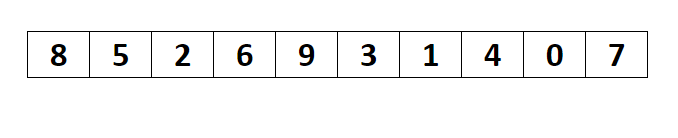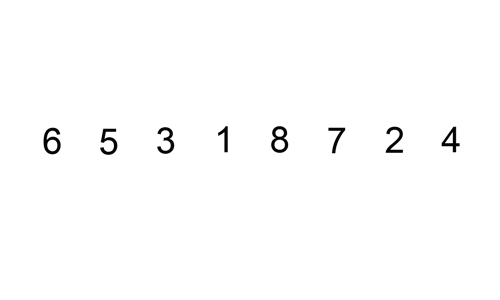# Sorting Algorithms

In today’s world, everyone has data, and we all want this data to look nice and organized. Besides our urge to see data in a well-ordered manner, a lot of the important algorithms are designed to work on sorted data. As you can imagine, it would be difficult to sort through data manually, and that’s why computers are used to perform these tasks. But sometimes, the data at hand can be enormous, and in these situations, even the most powerful computers can take a significant chunk of time to sort through data.

This is where the importance of the sorting algorithms comes to light. There are several sorting algorithms to choose from, each with its own benefits depending on the situation. With the right algorithm, you can sort data within a fraction of seconds which would have otherwise taken a considerable amount of time.

While choosing the algorithm, your data's initial condition comes into play as well; the array of elements could be random or reversed, it can be nearly sorted or sorted with a few unique values. When choosing an algorithm that most accurately fits your needs, you’ll have to become familiar with the different sorting algorithms used in practice.

Before we dive into the world of sorting algorithms, there are few mathematical notations you need to understand which are used for the asymptotic analysis of algorithms.

Don’t worry if you have heard this term for the first time. Soon we’ll see what exactly this means.

Prerequisites

Computing the exact runtime of an algorithm is a tedious task. It depends on knowing all kinds of fine details about how the program works and all kinds of fine details about how the computer works, how fast it is, what kind of system architecture it has. It’s a huge mess. We don’t want to go through this huge mess every single time we try to analyze an algorithm.

That’s why we use asymptotic analysis to measure the efficiency of an algorithm. This type of analysis helps us answer the question, how does our program’s runtime scale with the input size. 3 mathematical functions are used for calculating the asymptotic time complexity for an algorithm, Big O notation (worst case), Omega notation (best case), and Theta notation.

I’ll refrain from further discussing these topics here, as you are here to learn about sorting algorithms, and I don’t want to bore you with these mathematical functions.Taken from giphy.com

As of now, we’ll only use Big O notation, which represents the upper bound of the runtime of an algorithm. If you want to explore this topic, click here.

There are few more points that you should know

• What is a stable sorting algorithm? Algorithms that maintain the relative order of records with equal keys (values) are stable.
• What is an in-place sorting algorithm? Algorithms in which the sorted items occupy the same storage as the original ones are in place. This simply means there is no auxiliary space requirement for storing the sorted items

So armed with the knowledge of asymptotic analysis, and appreciating a few other terms, let us begin our journey exploring the various sorting algorithms, starting with the bubble sort.

Bubble Sort

• The bubble sort is the simplest sorting algorithm. Due to its simplicity, bubble sort is often used to introduce the concept of a sorting algorithm
• This algorithm works by swapping the adjacent elements time after time if they violate the sorted order.
• The average and worst-case time complexity of bubble sort is O(n*n), where the worst-case occurs when the input is reversely sorted
• Bubble sort can be optimized by stopping the algorithm if no swap occurred. This optimized version can be used to detect whether the input is already sorted, that too in a linear time!
• Bubble sort is a stable and in-place sorting algorithm

Selection Sort

• The selection sort works by finding the minimum element (considering ascending order) time after time from the unsorted part and places it at the beginning of the unsorted subarray.
• This algorithm maintains two subarrays in a given array. In first, the subarray is sorted. The second contains the remaining subarray.Taken from Coding Connect
• At each iteration of the selection sort, the unsorted part's minimum element is picked and moved to the sorted subarray.
• The worst and best case time complexity of selection sort is O(n*n), this happens because no matter what the input is, the algorithm will scan the array n times (n is the size) each time finding the minimum element which in turn takes n iterations
• Selection sort is an in-place algorithm. The default implementation of this algorithm is not stable. However, it can be made stable. For a more detailed explanation, click here
• An interesting thing about selection sort is it never makes more than n swaps (n is the size) and therefore can be useful when swapping operation is costly. Besides, it acts as a base for a more powerful sorting algorithm, the heap sort

Until now, the algorithms that we discussed were pretty basic, and that’s why I did not show you any practical implementation. From now, I’ll also include a code snippet (written in CPP) showing the practical implementation of the algorithms that we discuss. Though, I won’t be explaining the code here.

I encourage you to look at the description and the code snippet, then connect the dots yourself. Believe me, you’ll feel ecstatic and joyous after deciphering the underlying ideas behind these algorithms.

Insertion Sort

• Insertion sort is a sorting algorithm that moves an element to its right position at each iteration. The array is virtually broken into sorted and unsorted parts. Values from the unsorted subarray are picked and moved to the correct spot in the sorted subarray.Taken from Wikimedia Commons
• The worst-case time complexity of insertion sort is O(n*n), which occurs when the array is reversely sorted. The best-case (O(n)) occurs when the array is already sorted.
• Insertion sort is useful in situations where the size of the array is small or where the input is almost sorted. Besides, it also acts as a base for a more powerful sorting algorithm, the shell sort
• This is a stable and in-place sorting algorithm

I believe that’s enough algorithms for one day.

I hope you had a fun experience exploring these algorithms and enhanced your knowledge at the same time. If you wish to give feedback, feel free to contact me.

Resources